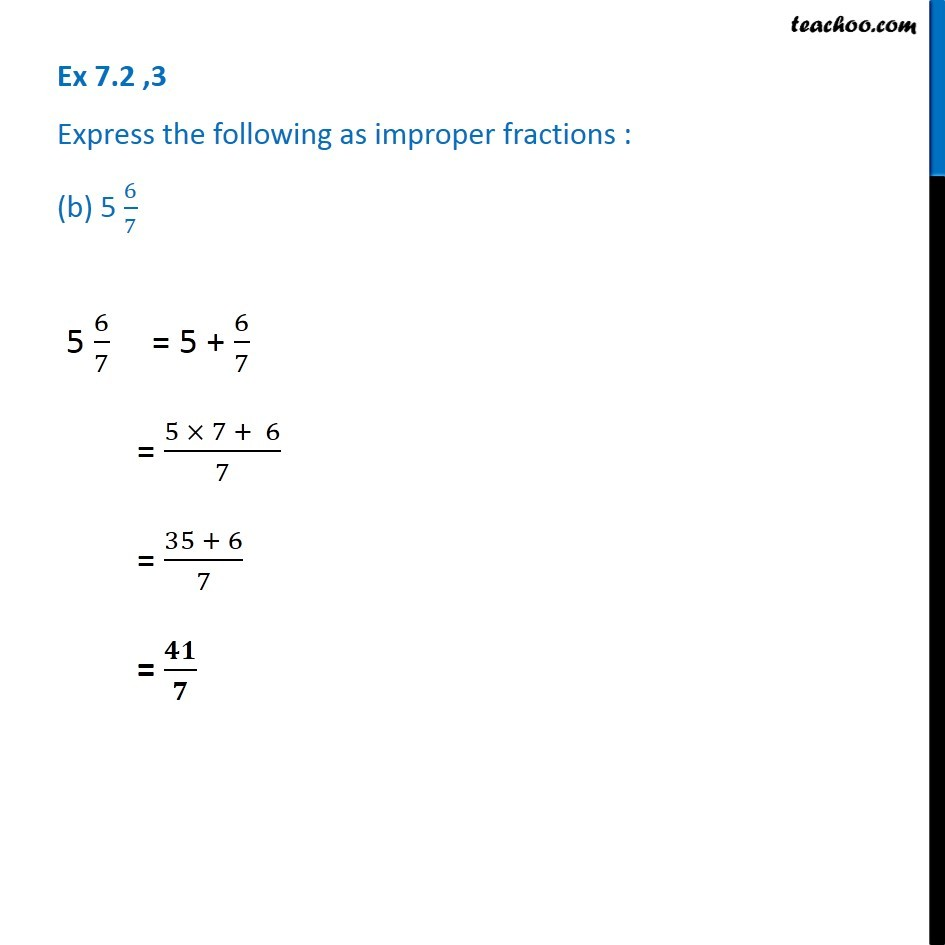1. Chapter 7 Class 6 Fractions
2. Serial order wise
3. Ex 7.2

Transcript

Ex 7.2 ,3 Express the following as improper fractions : (b) 5 6/7 5 6/7 = 5 + 6/7 = (5 × 7 + 6)/7 = (35 + 6)/7 = 𝟒𝟏/𝟕